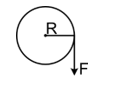Torque
Question

# A uniform disc of radius ‘R” and mass ‘M’ can rotate without friction on an axis passing through its centre and perpendicular to its plane. A cord is wound at the rim of the disc and a uniform force of F is applied on the cord. The tangential acceleration of a point on the rim of the disc is

Easy
Solution

##$\mathrm{FR}=\mathrm{I\alpha }=\frac{{\mathrm{MR}}^{2}}{2}\frac{\mathrm{a}}{\mathrm{R}}$$\mathrm{a}=\frac{2\mathrm{F}}{\mathrm{m}}$

Get Instant Solutions